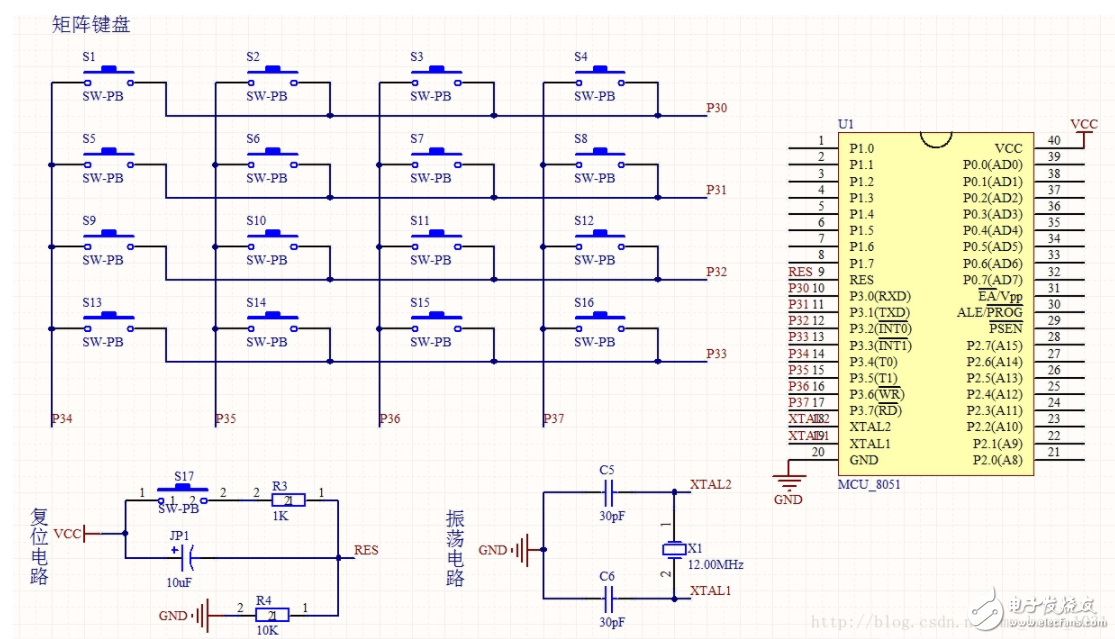51单片机矩阵键盘与8051连接设计#include《reg52.h》

unsigned char NUM=1;

/*-----------------------------

-----------------------------*/

sbit L1=P0^0;

sbit L2=P0^1;

sbit L3=P0^2;

sbit L4=P0^3;

sbit L5=P0^4;

sbit L6=P0^5;

sbit L7=P0^6;

sbit L8=P0^7;

sbit DUAN=P2^6;

sbit WEI=P2^7;

/*-----------------------------

-----------------------------*/

void init（）

{

EA=1;

ET0=1;

TMOD=0X01;

TH0=（65536-10000）/256;

TL0=（65536-10000）%256;

}

/*-----------------------------

-----------------------------*/

void TImer0（）interrupt 1

{

TR0=0; //定时终止

TH0=（65536-10000）/256; //定时器0初值重装

TL0=（65536-10000）%256;

NUM--;

}

/*--------------------------------------------------

--------------------------------------------------*/

unsigned char matrixkeyscan（）

{

unsigned char temp，reg1=0，reg2=0，key=0; //temp为临时变量，reg1为寄存器1，reg2为寄存器2，key为键盘编码号

P3=0x0f;

temp=P3&0x0f;

if（temp！=0x0f） //按键检测兼行坐标检测

{

TR0=1; //-------------------

while（NUM）; // 10ms去抖动延时

NUM=1; //-------------------

temp=P3&0x0f;

if（temp！=0x0f） //二次检测

{

reg1=temp; //把行坐标存入寄存器1

P3=reg1|0xf0; //关键，没有这句将导致整个函数出错

temp=P3&0xf0;

if（temp！=0xf0） //检测列坐标

{

reg2=temp; //把列坐标存入寄存器2

key=reg1|reg2; //将寄存器1和寄存器2进行按位或，作用是组合坐标，格式为八位二进制的“列坐标行坐标”

while（temp！=0xf0） //等待按键释放

temp=P3&0xf0;

}

}

}

return key;

}

/*--------------------------------------------------

--------------------------------------------------*/

void keyfuncTIon（）

{

unsigned char kvalue=0;

kvalue=matrixkeyscan（）;

switch（kvalue）

{

case 0xee： L1=~L1;/*按键01功能*/ break;

case 0xde： L2=~L2;/*按键02功能*/ break;

case 0xbe： L3=~L3;/*按键03功能*/ break;

case 0x7e： L4=~L4;/*按键04功能*/ break;

case 0xed： L5=~L5;/*按键05功能*/ break;

case 0xdd： L6=~L6;/*按键06功能*/ break;

case 0xbd： L7=~L7;/*按键07功能*/ break;

case 0x7d： L8=~L8;/*按键08功能*/ break;

case 0xeb： /*按键09功能*/ break;

case 0xdb： /*按键10功能*/ break;

case 0xbb： /*按键11功能*/ break;

case 0x7b： /*按键12功能*/ break;

case 0xe7： /*按键13功能*/ break;

case 0xd7： /*按键14功能*/ break;

case 0xb7： /*按键15功能*/ break;

case 0x77： /*按键16功能*/ break;

default： ;//空语句

}

}

/*-----------------------------

-----------------------------*/

void main（）

{

DUAN=0;

WEI=0;

init（）; //定时器初始化，装入初值10ms

while（1）

{

keyfuncTIon（）; //按键循环检测

}

}

• 没有资料